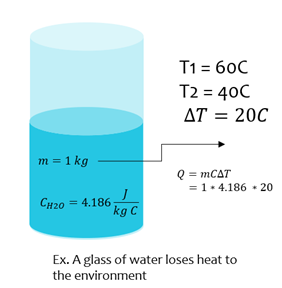# Top Chemistry Formulas

Here you will find the most common chemistry equations and formulas used in high school and fundamental university courses. These include, but are not limited to, temperature conversions, mole equations, pH, and ideal gas laws.

For more chemistry formulas visit Wikipedia.

## Basic Equations

### Atoms to Moles

A mole is an SI unit for measuring an amount of substance. The number of moles of anything can be calculated by taking the number of particles, (atoms, things, etc.) and dividing it by Avogadro’s number.

# Moles  =   (#Particles)  x
 1 mole 6.02 x 1023 particles### Fahrenheit to Celsius

Most chemistry equations use temperature in degrees C or Kelvins. If given temperature in F, you can convert to C with the following equation.

℃  =   (℉  -  32)  x
 5 9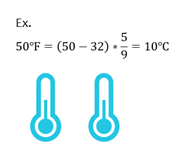### Celsius to Fahrenheit

If given temperature in C, you can convert to F with the following equation.

℉  =   (℃  x
 9 5
)  +  32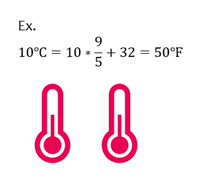### Celsius to Kelvins

If given temperature in C, you can convert to K with the following equation.

 K  =  ℃  +  273.15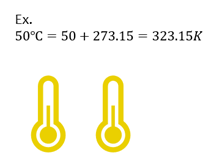## Solutions

### Molarity

Molarity is a measure of solution concentration which is calculated as the moles of solute per liter of solution.

 moles solute L solution

### Molality

Molality is a measure of solution concentration which is calculated as the moles of solute per kilogram of solvent.

 moles solute kg solvent

pH & pOH

pH  = - log⁡[H+]

pOH  =  - log log[OH-]

pH specifies the acidity or basicity of a solution, where [H+] means the concentration of hydrogen ions and [OH-] is the concentration of hydroxide ions.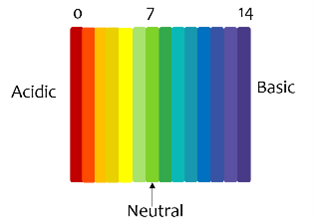### Henderson-Hasselbalch Acid Dissociation

Relationship between pH and pKa

pH  =  pKa  +
 [A-] [HA]
=  pKa  +
 [Base] [Acid]

## Atomic Structure

### DeBroglie

Particles can behave like waves. A wavelength can be given to a particle if the mass m and velocity v is known. h is Planck’s constant.

λ  =
 h mv

### Planck Einstein Relation

Where h is Planck’s constant

E  =  hf  =
 hc λ
c  =  λf

## Gases

### Boyle’s Law

Used when temperature is constant. P1 and V1 are the initial pressure and volume, and P2 and V2 are the new volume and pressure.

 P1V1  =  P2V2

### Charles’ Law

Used when pressure is constant. V1 and T1 are the initial volume and temperature, and T2 and V2 are the new temperature and pressure.

 V1 T1
=
 V2 T2

### Ideal Gas

Where R is the ideal gas constant

 PV  =  nRT

## Thermodynamics

### Heat Energy

Where m is mass of a substance (kg), c is the specific heat (J/kg∙K), and ∆T is change in temperature (Kelvins, K or Celsius, C)

 Q  =  mC ΔT